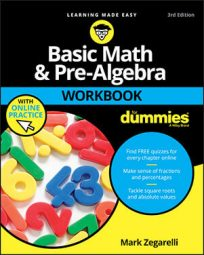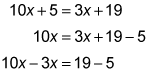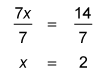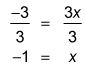##### Basic Math & Pre-Algebra Workbook For Dummies with Online PracticeA quick method for solving algebra problems is to re-arrange the equation by placing all x terms on one side of the equal sign and all constants (non-x terms) on the other side. Essentially, you're doing the addition and subtraction without showing it. You can then isolate x.

## Practice questions

1. Rearrange the equation 10x + 5 = 3x + 19 to solve for x.
2. Solve –[2(x + 7) + 1] = x – 12 for x.

1. x = 2First, rearrange the terms of the equation so that the x terms are on one side and the constants are on the other. In this case, you can do this in two steps:Second, combine like terms on both sides:

7x = 14

The third and final step is to divide (in this case, by 7) to isolate x:2. x = –1Before you can begin rearranging terms, remove the parentheses on the left side of the equation. Start with the inner parentheses, multiplying 2 by every term inside that set:

–[2(x + 7) + 1] = x – 12 –[2x + 14 + 1] = x – 12

Next, remove the remaining parentheses, switching the sign of every term within that set:

–2x – 14 – 1 = x – 12

Now you can solve for x by, first, rearranging the terms of the equation:

–2x – 14 – 1 + 12 = x –14 – 1 + 12 = x + 2x

Then you combine like terms on both sides:

–3 = 3x

Finally, you divide (in this example, by 3) to isolate x: